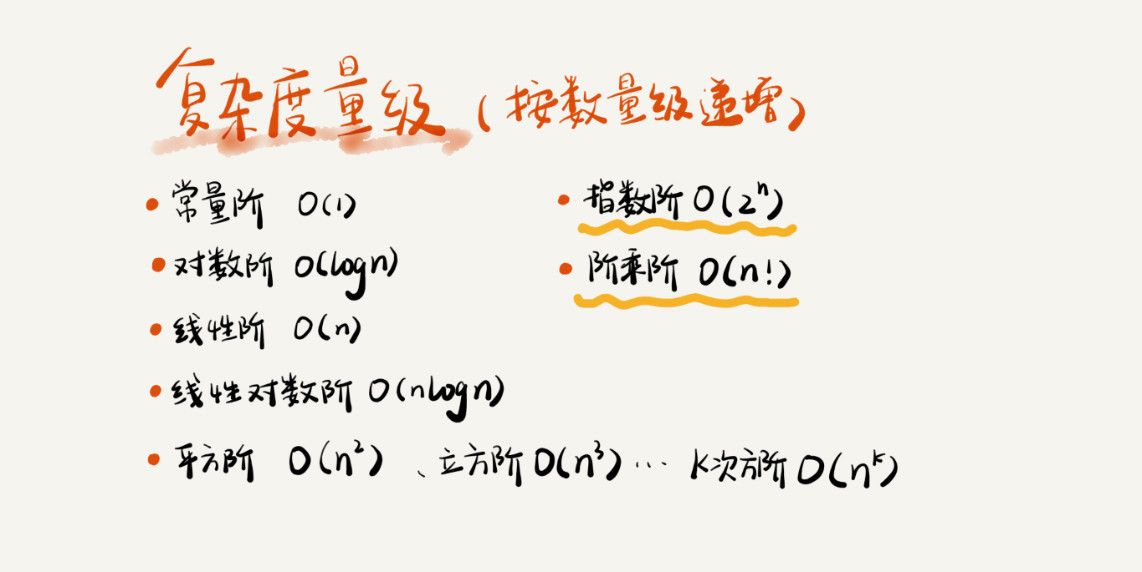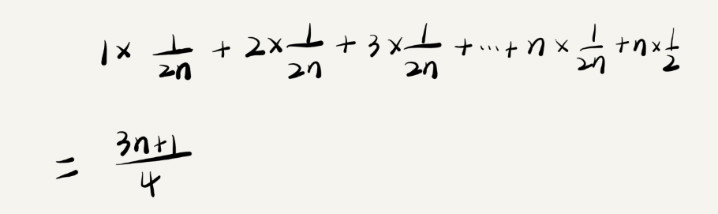#### 复杂度分析

2019-10-18 29979 ℃

## 时间复杂度

### 大O表示法• T(n)表示代码执行的时间
• n 表示数据规模的大小
• f(n) 表示每行代码执行的次数总和。因为这是一个公式，所以用 $f(n)$ 来表示
• O表示代码的执行时间 $T(n)$$f(n)$表达式成正比

### 时间复杂度分析

• 只关注循环执行次数最多的一段代码

 int cal(int n) {
int sum = 0;
int i = 1;
for (; i <= n; ++i) {
sum = sum + i;
}
return sum;
}


第 2、3 行代码都是常量级的执行时间，与 n 的大小无关，所以对于复杂度并没有影响。循环执行次数最多的是第 4、5 行代码，所以这块代码要重点分析。这两行代码被执行了 n 次，所以总的时间复杂度就是 $O(n)$

• 总复杂度等于量级最大的那段代码的复杂度

int cal(int n) {
int sum_1 = 0;
int p = 1;
for (; p < 100; ++p) {
sum_1 = sum_1 + p;
}

int sum_2 = 0;
int q = 1;
for (; q < n; ++q) {
sum_2 = sum_2 + q;
}

int sum_3 = 0;
int i = 1;
int j = 1;
for (; i <= n; ++i) {
j = 1;
for (; j <= n; ++j) {
sum_3 = sum_3 +  i * j;
}
}

return sum_1 + sum_2 + sum_3;
}



这个代码分为三部分，分别是求 sum_1、sum_2、sum_3。分别分析每一部分的时间复杂度，然后取一个量级最大的作为整段代码的复杂度。

这三段代码的时间复杂度分别是是 $O(1)$$O(n)$$O(n^2)$

综合这三段代码的时间复杂度，取其中最大的量级。所以，整段代码的时间复杂度就为$O(n^2)$

• 嵌套代码的复杂度等于嵌套内外代码复杂度的乘积

int cal(int n) {
int ret = 0;
int i = 1;
for (; i < n; ++i) {
ret = ret + f(i);
}
}

int f(int n) {
int sum = 0;
int i = 1;
for (; i < n; ++i) {
sum = sum + i;
}
return sum;
}



假设 f() 只是一个普通的操作，那第 4～6 行的时间复杂度就是，$T1(n) = O(n)$。但 f() 函数本身不是一个简单的操作，它的时间复杂度是 $T2(n) = O(n)$，所以，整个 cal() 函数的时间复杂度就是，$T(n) = T1(n) * T2(n) = O(n*n) = O(n^2)$

### 常见的复杂度量级

**多项式阶：**随着数据规模的增长，算法的执行时间和空间占用，按照多项式的比例增长。

如： $O(1)$（常数阶）、$O(logn)$（对数阶）、$O(n)$（线性阶）、$O(nlogn)$（线性对数阶）、O(n^2)（平方阶）、$O(n^3)$（立方阶）等

**非多项式阶：**随着数据规模的增长，算法的执行时间和空间占用暴增，这类算法性能极差。

如：$O(2^n)$（指数阶）、$O(n!)$（阶乘阶） 等## 空间复杂度

### 空间复杂度分析

void print(int n) {
int i = 0;
int[] a = new int[n];
for (i; i <n; ++i) {
a[i] = i * i;
}

for (i = n-1; i >= 0; --i) {
print out a[i]
}
}


## 特殊时间复杂度

### 最好最坏时间复杂度

// n 表示数组 array 的长度
int find(int[] array, int n, int x) {
int i = 0;
int pos = -1;
for (; i < n; ++i) {
if (array[i] == x) {
pos = i;
break;
}
}
return pos;
}



### 平均时间复杂度